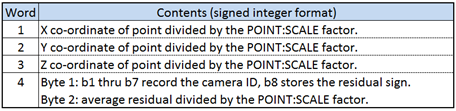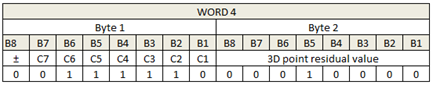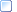﻿ 3D Data - Integer Format
 The 3D Point Data Section > Description

### 3D Data - Integer Format

If the POINT:SCALE parameter is positive then the 3D data section will contain signed integer format data for each stored trajectory.  Note that the POINT:SCALE parameter is one of the parameter section values that is copied to the C3D file header (words 7-8) and can be quickly located and read by software applications without requiring a detailed search of the parameter section.

The 3D integer point record is organized as follows:Figure 22 - 3D point data storage using signed integer format.

The first three signed integer words record the X, Y, and Z coordinate values of the 3D data point, divided by the floating-point POINT:SCALE parameter value.  Word four of the 3D point record is comprised of two bytes in the order determined by the endian format used by the C3D file.  The first byte stores which observers (normally cameras 1 through 7) provided information used in calculating the 3D point, while the second byte contains the average residual for the 3D point measurement.  The residual value (in POINT:UNITS) is a measurement of the accuracy of each point, although the calculation of the residual is performed by the photogrammetry software and may vary, depending of the software that generates the value.

The 3D point residual is a measurement that provides information about the relative accuracy of the 3D measurement and must be multiplied by the POINT:SCALE parameter to obtain a physical world scaled value.Figure 23 - Residual and mask storage - Integer format.

In the example shown above (big endian order) the word stored in the C3D file is 0x3E10h, indicating that cameras 2 through 6 have observed the point  and a residual value of 0x10h has been recorded which, multiplied by the stored POINT:SCALE factor of 0.0833333 (recorded in the parameter section), yields a residual of 1.333 for this observed point (0.0833333 * 16).

More:Notes for programmers - Integer 3D Data